﻿ 融合用户特征优化聚类的协同过滤算法
«上一篇文章快速检索 高级检索

 智能系统学报2020, Vol. 15Issue (6): 1091-1096  DOI: 10.11992/tis.2017100240

### 引用本文LIANG Lijun, LI Yegang, ZHANG Na’na, et al. Collaborative filtering algorithm combining user features and preferences in optimized clustering[J]. CAAI Transactions on Intelligent Systems, 2020, 15(6): 1091-1096. DOI: 10.11992/tis.201710024.### 文章历史

Collaborative filtering algorithm combining user features and preferences in optimized clustering
LIANG Lijun , LI Yegang, ZHANG Na’na , ZHANG Xiao , WANG Dong
College of Computer Science and Technology, Shandong University of Technology, Zibo 255049, China
Abstract: The collaborative filtering recommendation algorithm in the field of recommendation systems is still accompanied by the data sparsity, cold start, and scalability problems. To solve the cold start and scalability problems, we propose a PCEDS(pearson correlation coefficient and euclidean distance) collaborative filtering recommendation algorithm based on optimized clustering. First, the optimized K-means clustering algorithm is used to cluster the attributes of users. Then, based on the trust-based similarity model of user attribute features and the similarity model of user preference, a novel PCEDS similarity model is established to create a prediction model for the clustering results. The experimental results indicate that, compared with the traditional collaborative filtering recommendation algorithm, the proposed PCEDS collaborative filtering recommendation algorithm reduces the root mean square error by approximately 5%, significantly improves the recommendation precision and recall, and solves the cold start problem. Simultaneously, the clustering technology can save the memory space of the recommendation system, thereby improving its efficiency.
Key words: recommendation system    collaborative filtering    cold start    scalability    optimization clustering    trust degree    user attribute    user preference

1 基于用户活跃度(CF-act)聚类

K-means是聚类方法[12-13]中最常用的一种算法。在K-means算法中，初始聚类中心的选取对聚类结果有一定的影响。为了降低初始聚类中心对聚类结果的影响，本文在使用K-means聚类算法时，对初始聚类中心的选取进行了优化。年龄、性别、职业作为用户特有的特征，本文在对用户属性特征进行K-means聚类时，初始聚类中心即K值的选取，依据用户特有特征(年龄、性别、职业)进行K的选取，本文根据年龄段的划分，可以分为7个阶段，即K值为7。表 1 初始聚类中心 Tab.1 Initial clustering center

CUser表中选取n个用户，记为U={p1p2，…，pn}

q=0，i=0，j=0；

if q<3;

if pi[j]∈{0−17||18−24||25−34||35−44||45−49||50−55||56−}

then int sign:={0||1||2||3||4||5||6}

else if pi[j+1]∈{男|女}

then int sign:={0||1}

else if pi[j+2]∈{某一职业类}

then int sign:={0||1||2||3}

q++；i++；j++；

end if

end if

endDownload: 图 1 基于用户活跃度的K值 Fig. 1 K value based on user activity

initialize K, flag A={a1, a2, …, ak}

Repeat

For all uiU

For all bjB

bj∈max sim(ui,bj)

For all aiA

For all ujU

Until K no change

back

2 融合的相似度模型 2.1 基于加权用户属性特征和用户偏好的相似度模型

 $\mathop {{\rm{sim}}\left( {u,v} \right)}\nolimits^{{\rm{EDS}}} = \frac{1}{{1 + \sqrt {\displaystyle\sum\nolimits_{} {{{\left( {r_u - r_v} \right)}^2}} } }}$

 ${\rm{CD}}(u,v) = \frac{{\mathop {{\rm{ct}}}\nolimits_i }}{{\mathop {{\rm{ct}}}\nolimits_t }}$

 $\mathop {{\rm{sim}}\left( {u,v} \right)}\nolimits^{\rm CEDS} = \frac{{\mathop {{\rm{ct}}}\nolimits_i }}{{\mathop {{\rm{ct}}}\nolimits_t }} \frac{1}{{1 + \sqrt {\displaystyle\sum\nolimits_{} {{{\left( {r_u - r_v} \right)}^2}} } }}$

 $\mathop {{\rm{sim}}\left( {u,v} \right)}\nolimits^{{\rm{PCC}}} = \frac{{\displaystyle\sum\limits_{i \in I_{uv}} {\left( {r_{ui} - {\overline {r}}_{u} } \right)\left( {r_{vi} -{ \overline {r}}_{v} } \right)} }}{{\sqrt {\displaystyle\sum\limits_{i \in I_{uv}} {{{\left( {r_{ui} - {\overline {r}} _{u}} \right)}^2}{{\left( {r_{vi} - {\overline {r}}_{v} } \right)}^2}} } }}$
2.2 融合的2种相似度模型

 $\mathop {{\rm{sim}}\left( {u,v} \right)}\nolimits^{{\rm{PCEDS}}} = \lambda \times {\rm{PCC}} + (1 - \lambda )\times {\rm{CD}}\times {\rm{EDS}}$

3 实验结果与分析 3.1 PCEDS模型的RMSE结果

MovieLens数据集是做推荐系统最有权威的数据集，是一个涉及电影评分的真实数据集，由美国Minnesota大学提供。本文实验采用的是MovieLens 100 K数据集，评分数据的最高分为5，最低分为1，评分的高低代表用户的喜欢程度。在对用户进行聚类和CEDS模型计算相似度时采用的都是用户属性特征数据集，属性特征信息包括4个组：用户ID、年龄、性别、职业，其中职业包括21种职业类型。PCC模型计算相似度时采用的是Rating数据集，其信息包括用户ID、项目ID、评分。

 ${\rm{RMSE}}{\rm{ = }}\sqrt {\frac{{ \displaystyle\sum \limits_{i = 1}^N {{({p_i} - {q_i})}^2}}}{N}}$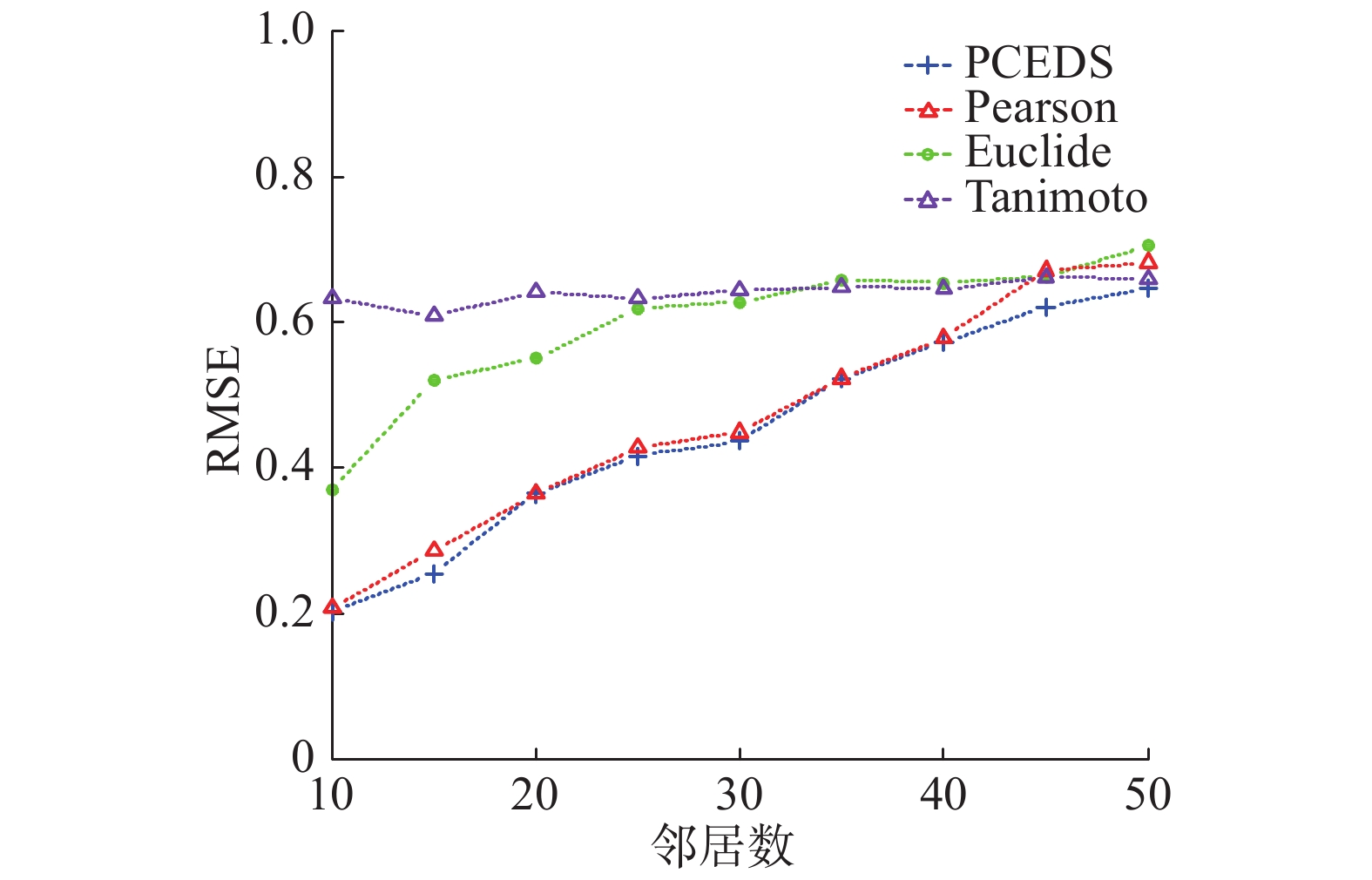Download: 图 2 PCEDS模型与Pearson、Euclide模型以及Tanimoto模型的RMSE Fig. 2 RMSE comparison between PCEDS、Pearson、Euclide and Tanimoto model

3.2 参数λ对PCEDS模型的影响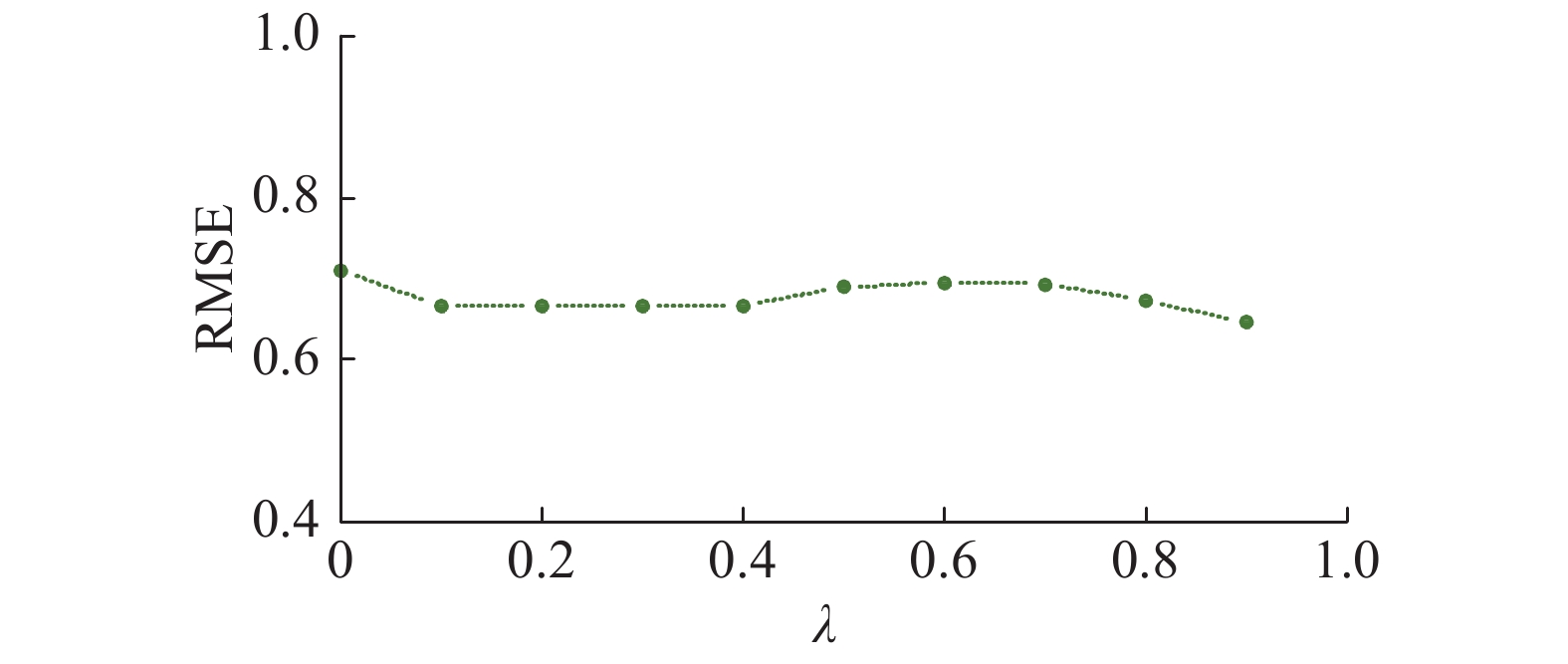Download: 图 3 λ变化对PCEDS模型的RMSE影响 Fig. 3 Effect of change on RMSE of PCEDS model
3.3 相融合的加权相似度模型(PCEDS)与PCC模型的precision/recall的比较

 $\begin{array}{*{20}{l}} {{\rm{Precision}} = \frac{{\displaystyle\sum\limits_u {|R(u) \cap T(u)|} }}{{\displaystyle\sum\limits_u {|R(u)|} }}}\\ {{\rm{Recall}} = \frac{{\displaystyle\sum\limits_u {|R(u) \cap T(u)|} }}{{\displaystyle\sum\limits_u {|T(u)|} }}} \end{array}$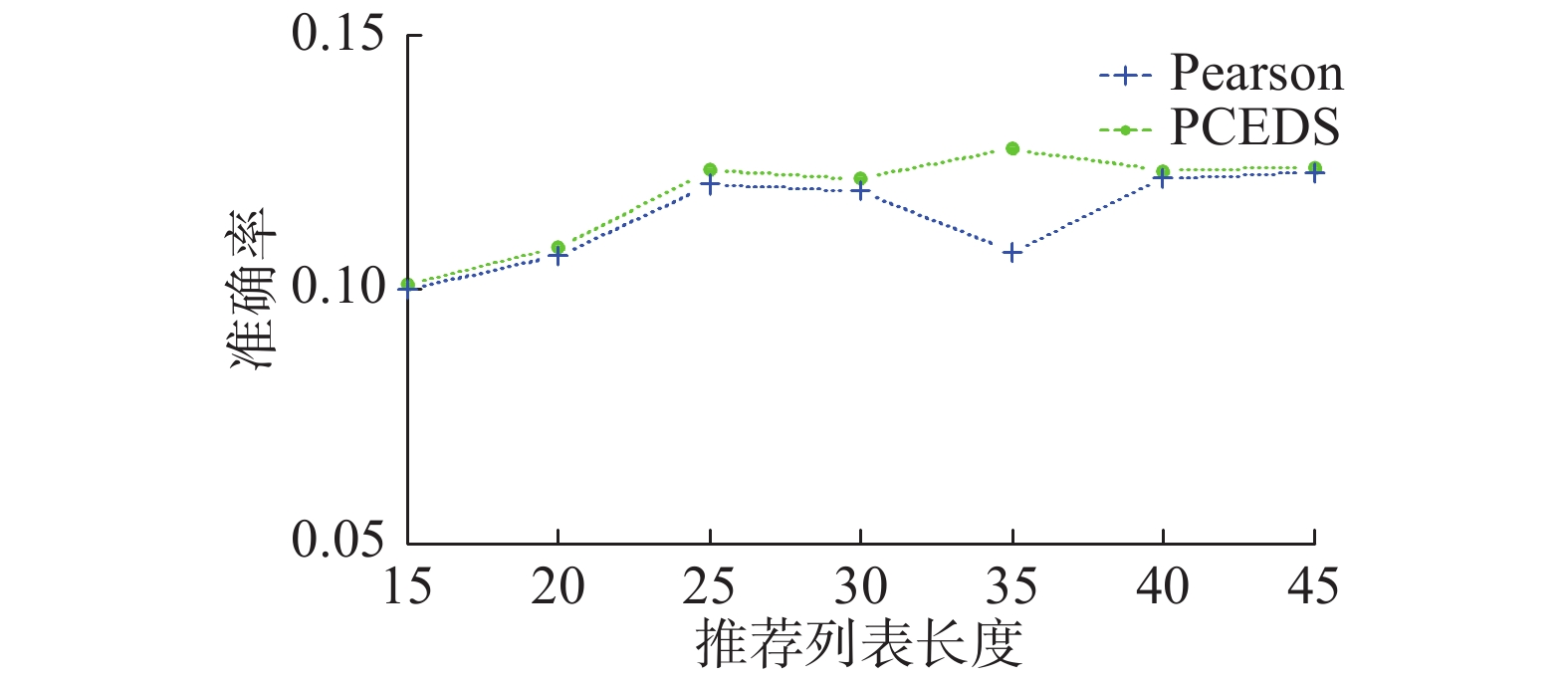Download: 图 4 准确率比较 Fig. 4 Comparison of precision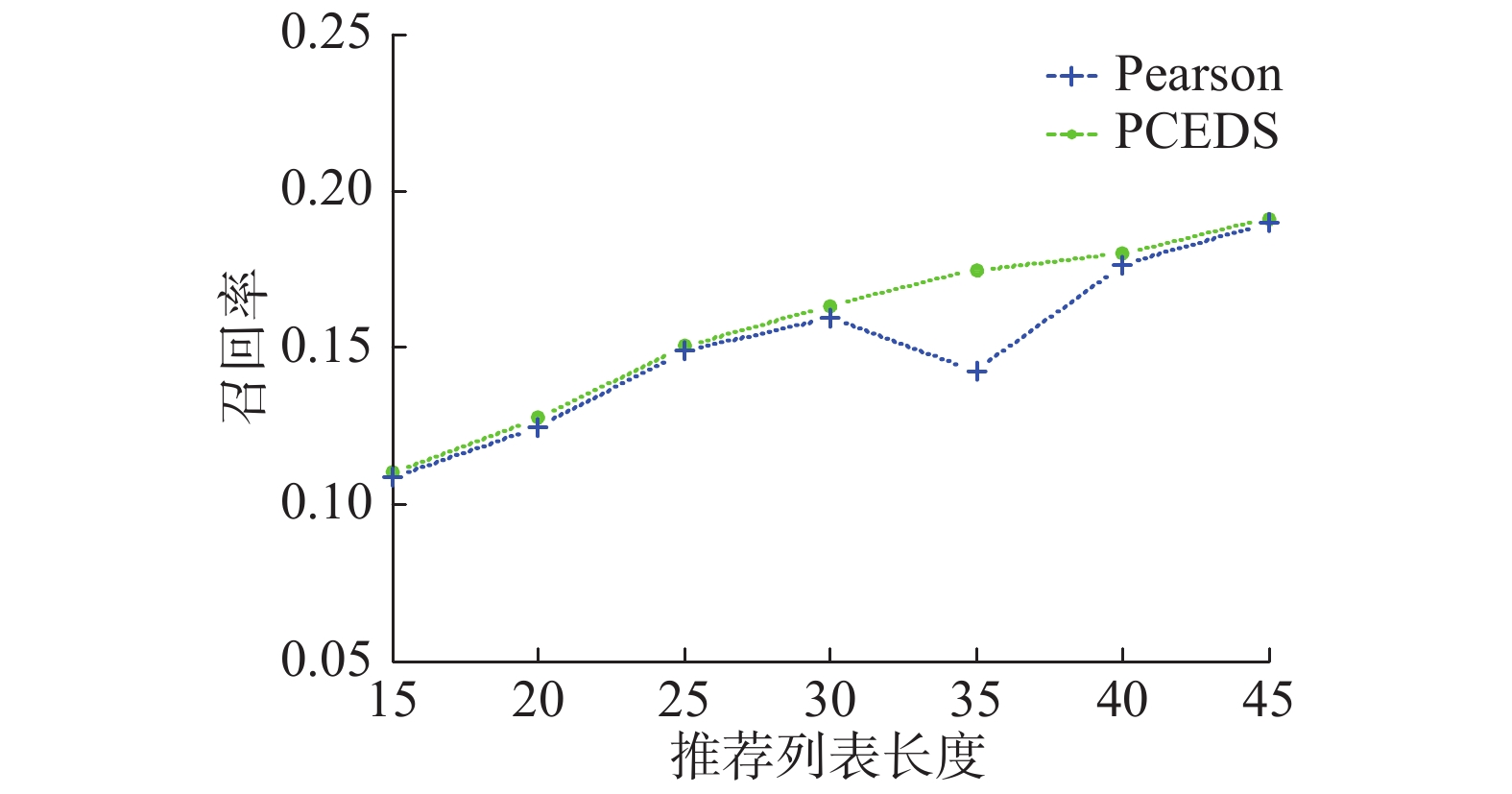Download: 图 5 召回率比较 Fig. 5 Comparison of recall

4 结束语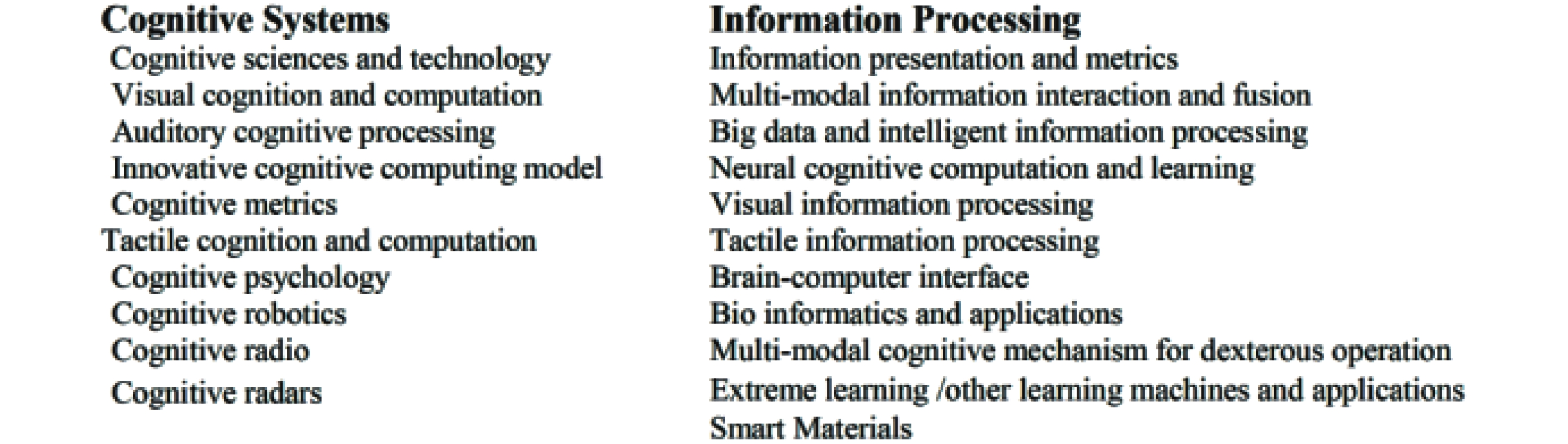Download: 图 1

  王国霞, 刘贺平. 个性化推荐系统综述[J]. 计算机工程与应用, 2012, 48(7): 66-76. WANG Guoxia, LIU Heping. Survey of personalized recommendation system[J]. Computer engineering and applications, 2012, 48(7): 66-76. DOI:10.3778/j.issn.1002-8331.2012.07.018 (0)  Jamali M, Ester M. A transitivity aware matrix factorization model for recommendation in social networks[C]//Proceedings of the Twenty-Second International Joint Conference on Artificial Intelligence. Barcelona, Spain,2011:2644–2649. (0)  WEI Jian, HE Jianhua, CHEN Kai, et al. Collaborative filtering and deep learning based recommendation system for cold start items[J]. Expert systems with applications, 2017, 69: 29-39. DOI:10.1016/j.eswa.2016.09.040 (0)  AGGARWAL C C. An introduction to data mining[M]//AGGARWAL C C. Data Mining: the Textbook. Cham: Springer, 2015: 1–26. (0)  孙天昊, 黎安能, 李明, 等. 基于Hadoop分布式改进聚类协同过滤推荐算法研究[J]. 计算机工程与应用, 2015, 51(15): 124-128. SUN Tianhao, LI Anneng, LI Ming, et al. Study on distributed improved clustering collaborative filtering algorithm based on Hadoop[J]. Computer engineering and applications, 2015, 51(15): 124-128. DOI:10.3778/j.issn.1002-8331.1405-0415 (0)  胡勋, 孟祥武, 张玉洁, 等. 一种融合项目特征和移动用户信任关系的推荐算法[J]. 软件学报, 2014, 25(8): 1817-1830. HU Xun, MENG Xiangwu, ZHANG Yujie, et al. Recommendation algorithm combing item features and trust relationship of mobile users[J]. Journal of software, 2014, 25(8): 1817-1830. (0)  LIU Haifeng, HU Zheng, MIAN A, et al. A new user similarity model to improve the accuracy of collaborative filtering[J]. Knowledge-based systems, 2014, 56: 156-166. DOI:10.1016/j.knosys.2013.11.006 (0)  韦素云, 肖静静, 业宁. 基于联合聚类平滑的协同过滤算法[J]. 计算机研究与发展, 2013, 50(S2): 163-169. WEI Suyun, XIAO Jingjing, YE Ning. Collaborative filtering algorithm based on co-clustering smoothing[J]. Journal of computer research and development, 2013, 50(S2): 163-169. (0)  LEE D D, SEUNG H S. Learning the parts of objects by non-negative matrix factorization[J]. Nature, 1999, 401(6755): 788-791. DOI:10.1038/44565 (0)  ELKAHKY A M, SONG Yang, HE Xiaodong. A multi-view deep learning approach for cross domain user modeling in recommendation systems[C]//Proceedings of the 24th International Conference on World Wide Web. Florence, Italy, 2015: 278-288. (0)  陈克寒, 韩盼盼, 吴健. 基于用户聚类的异构社交网络推荐算法[J]. 计算机学报, 2013, 36(2): 349-359. CHEN Kehan, HAN Panpan, WU Jian. User clustering based social network recommendation[J]. Chinese journal of computers, 2013, 36(2): 349-359. (0)  张顺龙, 库涛, 周浩. 针对多聚类中心大数据集的加速K-means聚类算法[J]. 计算机应用研究, 2016, 33(2): 413-416. ZHANG Shunlong, KU Tao, ZHOU Hao. Accelerate K-means for multi-center clustering of big datasets[J]. Application research of computers, 2016, 33(2): 413-416. DOI:10.3969/j.issn.1001-3695.2016.02.021 (0)  贾洪杰, 丁世飞, 史忠植. 求解大规模谱聚类的近似加权核K-means算法[J]. 软件学报, 2015, 26(11): 2836-2846. JIA Hongjie, DING Shifei, SHI Zhongzhi. Approximate weighted kernel K-means for large-scale spectral clustering[J]. Journal of software, 2015, 26(11): 2836-2846. (0)  DRAISMA J, HOROBET E, OTTAVIANI G, et al. The Euclidean distance degree of an algebraic variety[J]. Foundations of computational mathematics, 2016, 16(1): 99-149. DOI:10.1007/s10208-014-9240-x (0)